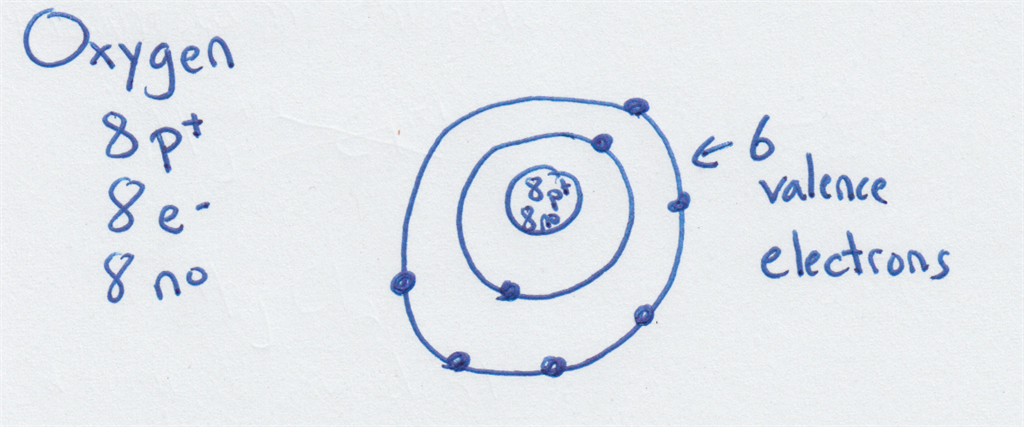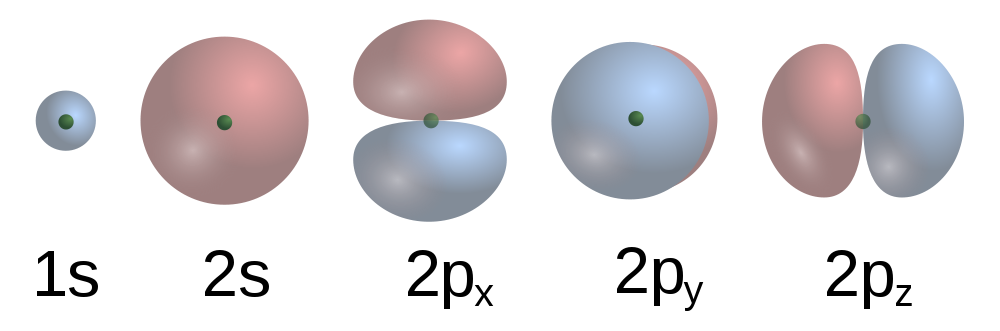# draw your own bohr model for dickson onlymdsbro
Atomic models are useful because they allow us to picture what is inside of an atom, something we will never be able to actually see. We're going to talk about two models, the Bohr model and the Quantum model. While we know that the Quantum model is the correct way to represent atoms, the Bohr model is still useful for a very basic understanding. Think of it as a stick figure sketch of an atom. The parts are all there, its just not quite filled in all of the way.

Bohr Model
The Bohr model of the atom was developed in 1914 by Niels Bohr. In this model, electrons move around the nucleus in fixed, concentric circles. Picture the planets orbiting the Sun. These circles are called energy levels and electrons must have a specific amount of energy to be in each level. The energy levels closest to the nucleus require the least amount of energy. In order for an electron to move further from the nucleus (or move up an energy level) they must gain energy. If they lose energy they fall down to an energy level closer to the nucleus.Drawing the Bohr model for an atom gives you valuable information about the atom's valence electrons. The valence electrons--those in the outermost energy level--are the ones that determine the chemical properties of an atom. In order to draw a Bohr model you must first use the periodic table to tell you the number of protons, neutrons, and electrons in an atom. For Bohr models we usually draw what the "average" atom for that element looks like and just round the average atomic mass to a whole number and use that for the mass number.

Steps for Drawing a Bohr Model:
1. Count the number of protons, electrons, and neutrons for that atom.
2. Draw the nucleus. Indicate the correct number of protons and neutrons in the nucleus.
3. Draw energy levels around the nucleus, starting with the energy level closest to the nucleus. Fill up each energy level before moving on to the next.

Each energy level in a Bohr model can only hold a specific number of electrons, as shown in the table below.
 Energy Level Max # of electrons 1 2 2 8 3 18 4 32

For example, here is a Bohr model for the average oxygen atom:Here is one for phosphorus:You try drawing one for Magnesium (Mg) and for Boron (B). Count the number of electrons on the outermost energy level to find the number of valence electrons. Check your answers by looking at the handout.

Quantum Model
In 1925 the Quantum model of the atom was developed after it was determined that electrons can behave like a wave and a particle at the same time. In addition, you can't know the precise location of an electron. Instead of traveling in orderly circles around the nucleus, we describe the electrons as existing in a three-dimensional electron cloud--a shape surrounding the nucleus. You will learn more about the Quantum model in chemistry.These are some of the orbital shapes possible in the first two energy levels of an atom.

Draw a Bohr model for elements with atomic numbers 1-18 (Hydrogen thru Argon). Draw each diagram neatly, clearly labeling the correct number of protons, electrons, and neutrons for each diagram. Use the average atomic mass from the periodic table for the mass number (round to the nearest whole number). These can be drawn by hand and scanned or photographed, or can be drawn electronically. Count and label the valence electrons (those in the outermost energy level) for each diagram. Upload your diagrams and the answers to the two questions below here.

Questions:
1. Look at your diagrams for hydrogen, lithium, and sodium. What do they all have in common? What group are these elements in on the periodic table?

2. Look at your diagrams for fluorine and chlorine. What do they have in common?

• 8 years ago
• 3
•